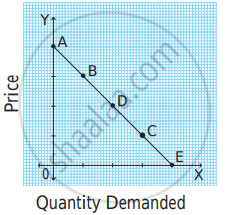# In the following diagram, AE is the linear demand curve of a commodity. On the basis of the given diagram state whether the following statement is True or False. - Economics

MCQ
True or False

In the following diagram, AE is the linear demand curve of a commodity. On the basis of the given diagram state whether the following statement is True or False. Give a reason for your answer.Demand at point ‘C’ is relatively elastic demand.

• True

• False

#### Solution

False.

Correct Statement: Demand at point ‘C’ is a relatively inelastic demand.

Reason: The length of the lower segment is less as compared to the upper segment at point C. Therefore, Ed < 1. Thus, at point C, demand is relatively inelastic.

Concept: Types of Price Elasticity of Demand
Is there an error in this question or solution?

#### APPEARS IN

Balbharati Economics 12th Standard HSC Maharashtra State Board
Chapter 3.2 Elasticity of Demand
Exercise | Q 7. (2)(1) | Page 36